# MA.912.GR.1.5Export Print
Prove relationships and theorems about trapezoids. Solve mathematical and real-world problems involving postulates, relationships and theorems of trapezoids.

### Clarifications

Clarification 1: Postulates, relationships and theorems include the Trapezoid Midsegment Theorem and for isosceles trapezoids: base angles are congruent, opposite angles are supplementary and diagonals are congruent.

Clarification 2: Instruction includes constructing two-column proofs, pictorial proofs, paragraph and narrative proofs, flow chart proofs or informal proofs.

Clarification 3: Instruction focuses on helping a student choose a method they can use reliably.

General Information
Subject Area: Mathematics (B.E.S.T.)
Strand: Geometric Reasoning
Status: State Board Approved

## Benchmark Instructional Guide

### Terms from the K-12 Glossary

• Angle
• Parallelogram
• Rectangle
• Rhombus
• Square
• Supplementary Angles
• Trapezoid

### Vertical Alignment

Previous Benchmarks

Next Benchmarks

### Purpose and Instructional Strategies

In elementary grades, students identified and classified trapezoids. In grade 7, students solved problems involving the area of trapezoids. In Geometry, students establish relationships between sides, angles and diagonals of trapezoids (including the special cases of trapezoids: parallelograms, rectangles, rhombi and squares), prove the theorems related to these relationships and use them to solve mathematical and real-world problems. In later courses, students will use trapezoids in cases like determining the area under a curve.
• Relationships, postulates and theorems in this benchmark should focus on, but are not limited to, the ones stated in Clarification 1. Additionally, some postulates and theorems have a converse (i.e., if conclusion, then hypothesis) that can be included.
• Instruction includes the connection to the Logic and Discrete Theory benchmarks when developing proofs. Additionally, with the construction of proofs, instruction reinforces the Properties of Operations, Equality and Inequality. (MTR.5.1
• Instruction utilizes different ways students can organize their reasoning by constructing various proofs when proving geometric statements. It is important to explain the terms statements and reasons, their roles in a geometric proof, and how they must correspond to each other. Regardless of the style, a geometric proof is a carefully written argument that begins with known facts, proceeds from there through a series of logical deductions, and ends with the statement you are trying to prove. (MTR.2.1
• For examples of different types of proofs, please see MA.912.LT.4.8
• Instruction includes the connection to compass and straight edge constructions and how the validity of the construction is justified by a proof. (MTR.5.1
• Students should develop an understanding for the difference between a postulate, which is assumed true without a proof, and a theorem, which is a true statement that can be proven. Additionally, students should understand why relationships and theorems can be proven and postulates cannot.
• Instruction includes the use of hatch marks, hash marks, arc marks or tick marks, a form of mathematical notation, to represent segments of equal length or angles of equal measure in diagrams and images.
• Students should understand the difference between congruent and equal. If two segments are congruent (i.e., PQMN), then they have equivalent lengths (i.e., PQ = MN) and the converse is true. If two angles are congruent (i.e., ∠ABC ≅ ∠PQR), then they have equivalent angle measure (i.e., $m$ABC = $m$PQR) and the converse is true.
• Instruction includes the use of hands-on manipulatives and geometric software for students to explore relationships, postulates and theorems.
• The K-12 Mathematics Glossary defines trapezoids as a quadrilateral with at least one pair of parallel sides (called the inclusive definition of trapezoid), therefore, all parallelograms are trapezoids.
• The Trapezoid Midsegment Theorem states that a line segment connecting the midpoints of the legs of the trapezoid, called midsegment, is parallel to the bases, and equal to half their sum (or the average of the length of the bases). It is important to introduce and discuss the new vocabulary related to trapezoids: legs (the two non-parallel sides) and bases (the two parallel sides).
• When proving the Trapezoid Midsegment Theorem, instruction includes the connection to coordinate geometry or to triangle congruence and the Triangle Midsegment Theorem.
• For example, given trapezoid ABCD on the coordinate plane with A at the origin, B at the point ($b$, 0), $c$ at point ($x$, $y$) and D at the point ($x$ + $d$, $y$), students can determine the midpoint of AC at the point P, ($\frac{\text{1}}{\text{2}}$$x$, $\frac{\text{1}}{\text{2}}$$y$) and the midpoint of BD at the point Q, ($\frac{\text{x+b+d}}{\text{2}}$, $\frac{\text{1}}{\text{2}}$$y$). Students should realize that PQ is horizontal and parallel to the base of the trapezoid, AB. To determine the length of PQ, students can subtract the $x$-coordinates of P and Q to find it has a length of $\frac{\text{1}}{\text{2}}$($b$ + $d$). Since PQ = $\frac{\text{1}}{\text{2}}$($b$ + $d$), AB = $b$ and CD = $d$, then PQ = $\frac{\text{1}}{\text{2}}$(AB + CD).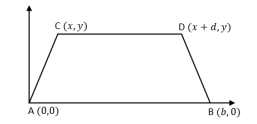• Instruction includes the understanding that an isosceles trapezoid is a special case of trapezoid where the legs are congruent and base angles are congruent. Properties of isosceles trapezoids include opposite angles are supplementary and diagonals are congruent. When proving properties of isosceles trapezoids, students can use triangle congruence or relationships between angles measures of parallel lines cut by a transversal (MTR.3.1).
• For example, given isosceles trapezoid ABCD and its diagonals, AC and BD, students can prove that the diagonals are congruent, AC ≅ DB, by proving that ΔADC ≅ ΔBCD. Students should be able to realize that they can use Side-Angle-Side (SAS) to prove that ΔADC ≅ ΔBCD. Then, students can conclude that ACDB.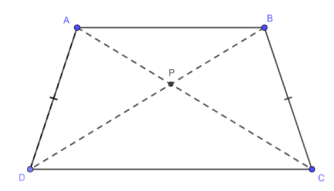• Instruction includes discussing that if a trapezoid also happens to be a parallelogram, then one can decide which side is to be called the base. Students can choose any of the four sides.
• Within Clarification 1, it is important to note that that having congruent base angles is not a consequence of a definition, but rather part of the definition.

### Common Misconceptions or Errors

• Students may think that all trapezoids are isosceles trapezoids. To help address this misconception, include examples of different types of trapezoids such as scalene trapezoids, right trapezoids and trapezoids where the parallel sides are not horizontal or vertical.
• Students might think all parallelograms are not trapezoids. To help address this misconception, reiterate the definition of a trapezoid (quadrilateral with at least one pair of parallel sides). Additionally, have students discuss special cases of trapezoids (e.g., isosceles and right) and why they are or are not considered parallelograms.

• Trapezoid JKLM is graphed on a coordinate plane.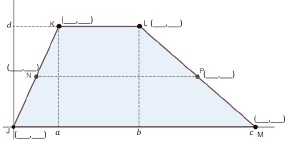• Part A. What are the coordinates of points J, K, L and M
• Part B. N is the midpoint of segment JK and P is the midpoint of segment LM. What are the coordinates of points N and P
• Part C. What are the lengths of segments KL, JK and NP
• Part D. Use your answers from Parts A through C to prove the Trapezoid Midsegment Theorem.

• Isosceles trapezoid ABCD is shown.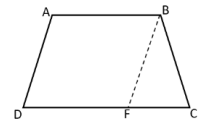• Part A. Prove that ∠A is supplementary to ∠D and that ∠B is supplementary to ∠C
• Part B. Prove that ∠A ≅ ∠B
• Part C. Prove that ∠A is supplementary to ∠C and that ∠B is supplementary to ∠D
• Part D. What do you know about isosceles trapezoid ABCD based on the proofs from Parts A to C?

• Quadrilateral ABCD is shown with a base that is parallel to its opposite side and has a pair of non-parallel sides. Assume that AD || BF.
• Part A. Prove that if non-parallel sides are congruent, then triangle BFC is an isosceles triangle.
• Part B. Prove that if the base angles, ∠C and ∠D, are congruent, then triangle BFC is an isosceles triangle.
• Part C. Prove that the base angles are congruent if and only if the non-parallel opposite sides are congruent.
• Part D. Classify the quadrilateral.

### Instructional Items

Instructional Item 1
• Tulips should be planted three inches apart to give a full look. The Starlings have a trapezoidal plot for a flower garden, as shown in the figure. They are going to put tulips along the parallel sides of the garden. The midsegment to the garden is 10 feet long. Tulips are sold in bags of 25 bulbs.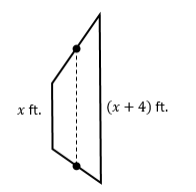• Part A. What are the lengths of the parallel sides of the garden?
• Part B. How many tulips are needed to line the parallel sides?
• Part C. What is the minimum number of bags the Starlings need to purchase to have enough bulbs to line the parallel sides of the garden?

*The strategies, tasks and items included in the B1G-M are examples and should not be considered comprehensive.

## Related Courses

This benchmark is part of these courses.
1200400: Foundational Skills in Mathematics 9-12 (Specifically in versions: 2014 - 2015, 2015 - 2022, 2022 and beyond (current))
1206310: Geometry (Specifically in versions: 2014 - 2015, 2015 - 2022, 2022 and beyond (current))
1206320: Geometry Honors (Specifically in versions: 2014 - 2015, 2015 - 2022, 2022 and beyond (current))
1206315: Geometry for Credit Recovery (Specifically in versions: 2014 - 2015, 2015 - 2022, 2022 and beyond (current))
7912065: Access Geometry (Specifically in versions: 2015 - 2022, 2022 and beyond (current))

## Related Access Points

Alternate version of this benchmark for students with significant cognitive disabilities.
MA.912.GR.1.AP.5: Use the relationships and theorems about trapezoids. Solve mathematical and/or real-world problems involving postulates, relationships and theorems of trapezoids.

## Related Resources

Vetted resources educators can use to teach the concepts and skills in this benchmark.

## Formative Assessment

An Isosceles Trapezoid Problem:

Students are asked to explain why the sum of the lengths of the diagonals of an isosceles trapezoid is less than its perimeter.

Type: Formative Assessment

## MFAS Formative Assessments

An Isosceles Trapezoid Problem:

Students are asked to explain why the sum of the lengths of the diagonals of an isosceles trapezoid is less than its perimeter.

## Student Resources

Vetted resources students can use to learn the concepts and skills in this benchmark.

## Parent Resources

Vetted resources caregivers can use to help students learn the concepts and skills in this benchmark.